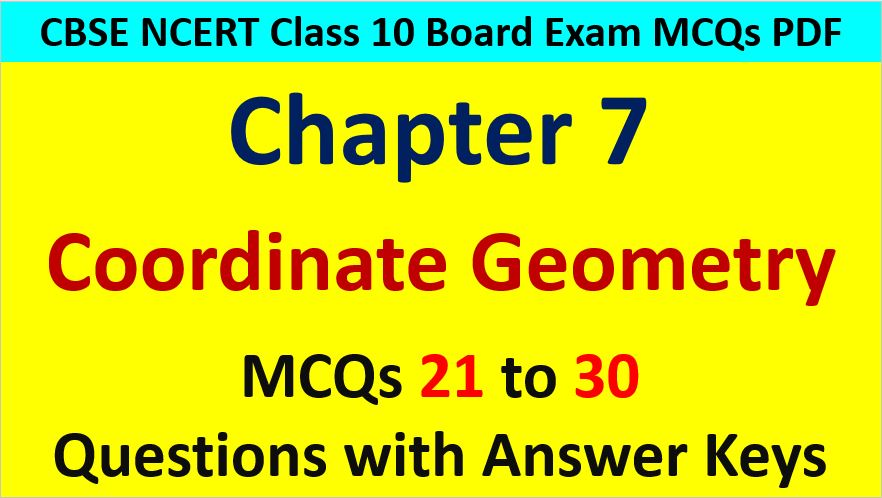Friday, October 22, 2021
Home > CBSE Class 10 > Important MCQ Questions for Class 10 Maths Chapter 7 Coordinate Geometry

# Important MCQ Questions for Class 10 Maths Chapter 7 Coordinate GeometryHi students, Welcome to AMBiPi (Amans Maths Blogs). In this article, you will get Important MCQ Questions for Class 10 Maths Chapter 7 Coordinate Geometry. You can download this PDF and save it in your mobile device or laptop etc.

Important MCQ Questions of Coordinate Geometry Class 10 Question No 21:

The distance of the points A(0, 6) and B(0, -2) is

Option A : 6

Option B : 8

Option C : 4

Option D : 2

Option B : 8

Important MCQ Questions of Coordinate Geometry Class 10 Question No 22:

The distance of the point (4, 7) from the y-axis is

Option A : 4

Option B : 7

Option C : 11

Option D : √65

Option A : 4

Important MCQ Questions of Coordinate Geometry Class 10 Question No 23:

The distance of the point P(2, 3) from the x-axis is

Option A : 2

Option B : 3

Option C : 1

Option D : 5

Option B : 3

Important MCQ Questions of Coordinate Geometry Class 10 Question No 24:

If the distance between the points A(2, -2), B(-1, x) is 5. Then, x is

Option A : -2

Option B : 2

Option C : -1

Option D : 1

Option B : 2

Important MCQ Questions of Coordinate Geometry Class 10 Question No 25:

The distance of the point (α, β) from the origin is

Option A : α + β

Option B : α2 + β2

Option C : |α| + |β|

Option D : √(α2 + β2)

Option D : √(α2 + β2)

Important MCQ Questions of Coordinate Geometry Class 10 Question No 26:

Point A is on y-axis at a distance 4 units from origin. If coordinates of point B are (-3, 0). The length of AB is

Option A : 7 units

Option B : 5 units

Option C : 49 units

Option D : 25 units

Option B : 5 units

Important MCQ Questions of Coordinate Geometry Class 10 Question No 27:

If A and B are points (-6, 7) and (-1, -5) respectively, then the distance 2AB is

Option A : 13

Option B : 26

Option C : 169

Option D : 238

Option B : 26

Important MCQ Questions of Coordinate Geometry Class 10 Question No 28:

A(2, 2), B(-2, 4), C(-2, 6) are vertices of a triangle ABC. Then, the triangle ABC is

Option A : Equilateral

Option BScalene

Option C : Isosceles

Option D : None of these

Option B : Scalene

Important MCQ Questions of Coordinate Geometry Class 10 Question No 29:

The mid points of line segment joining the points A(-2, 8) and B(-6, -4) is

Option A : (-4, -6)

Option B : (2, 6)

Option C : (-4, 2)

Option D : (4, 2)

Option C : (-4, 2)

Important MCQ Questions of Coordinate Geometry Class 10 Question No 30:

The coordinate of point P, where PQ is the diameter of the circle whose center is (2, -3) and the coordinate of Q is (1, 4), is

Option A : (3, -10)

Option B : (2, -10)

Option C : (-3, 10)

Option D : (-2, 10)